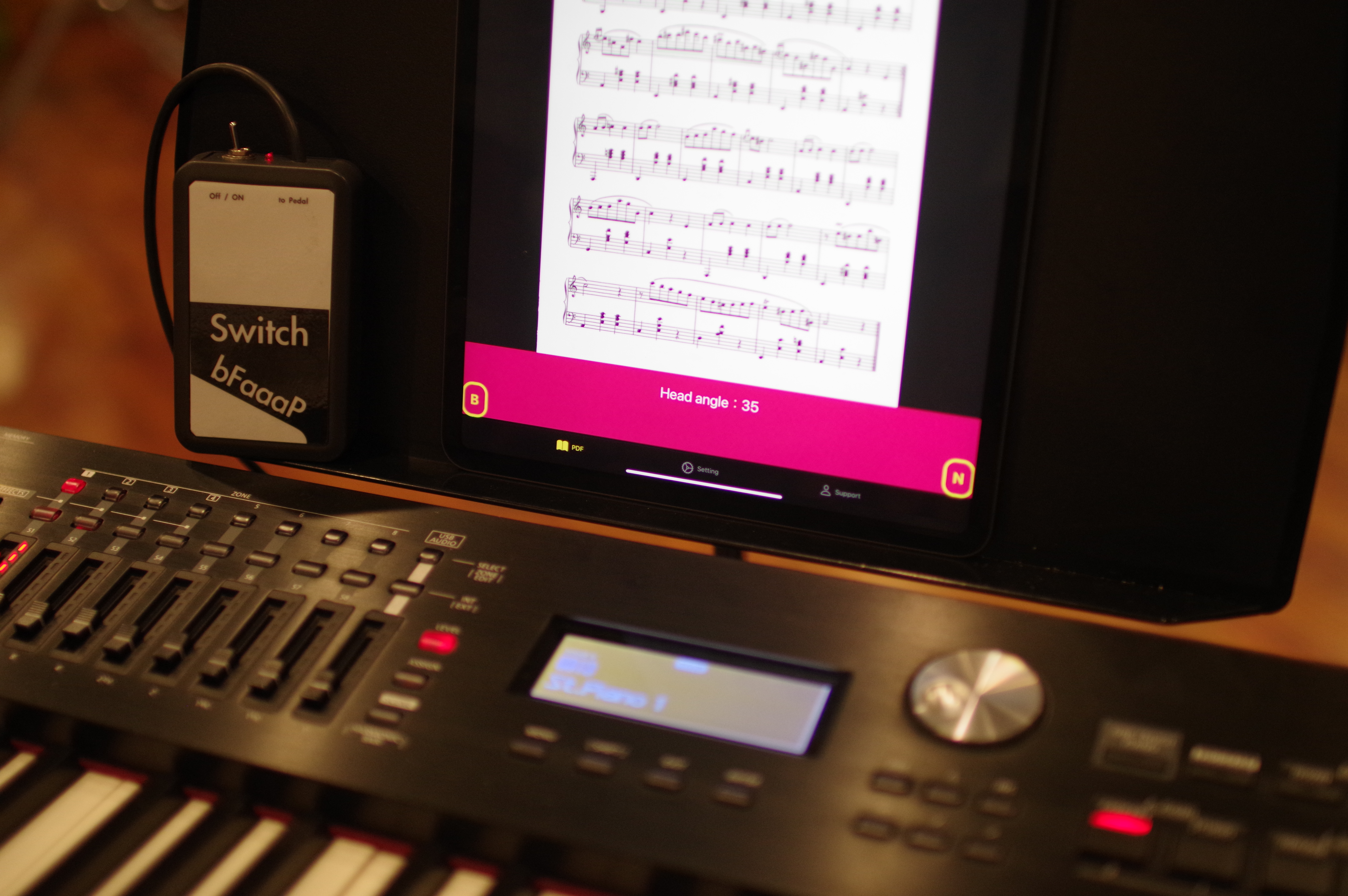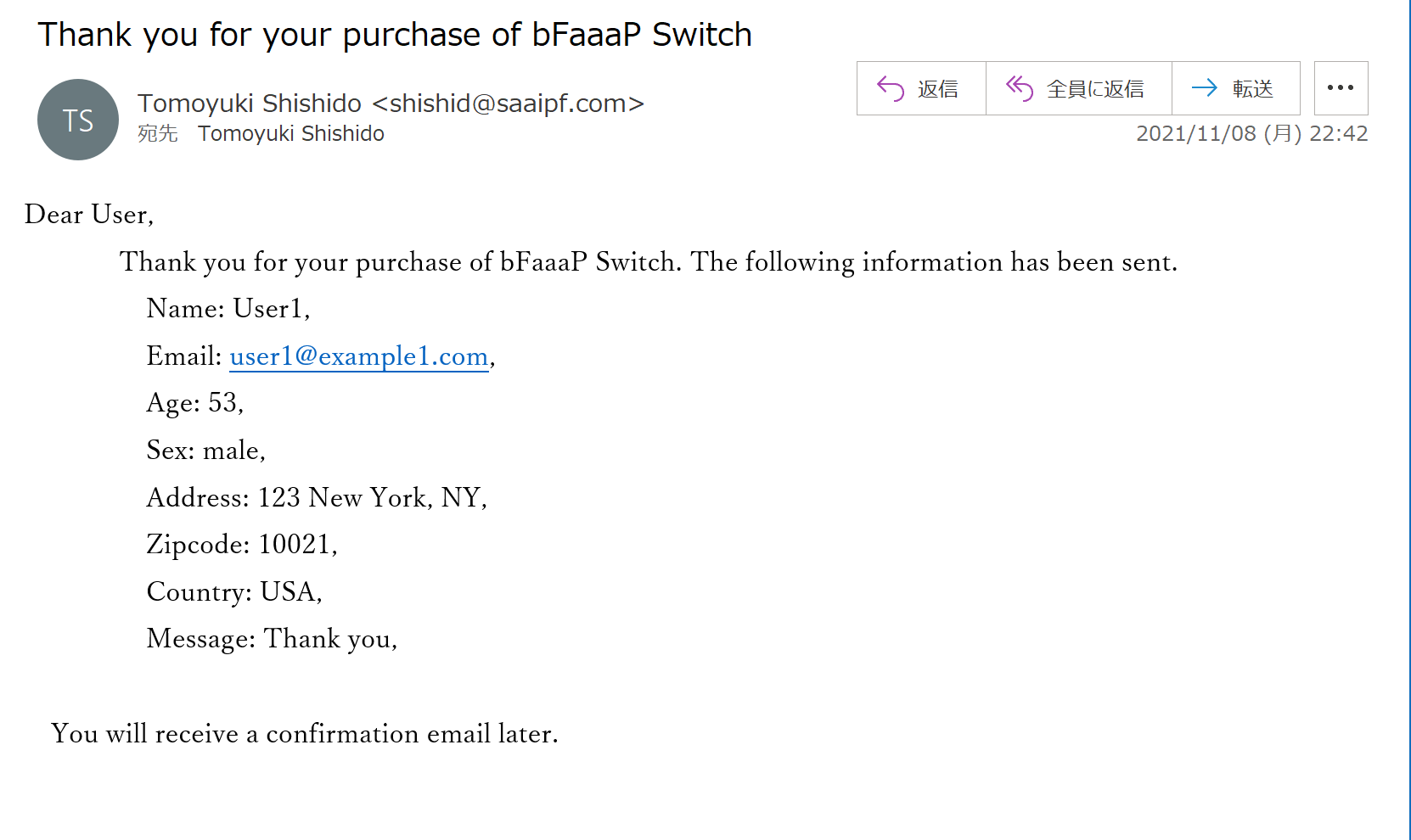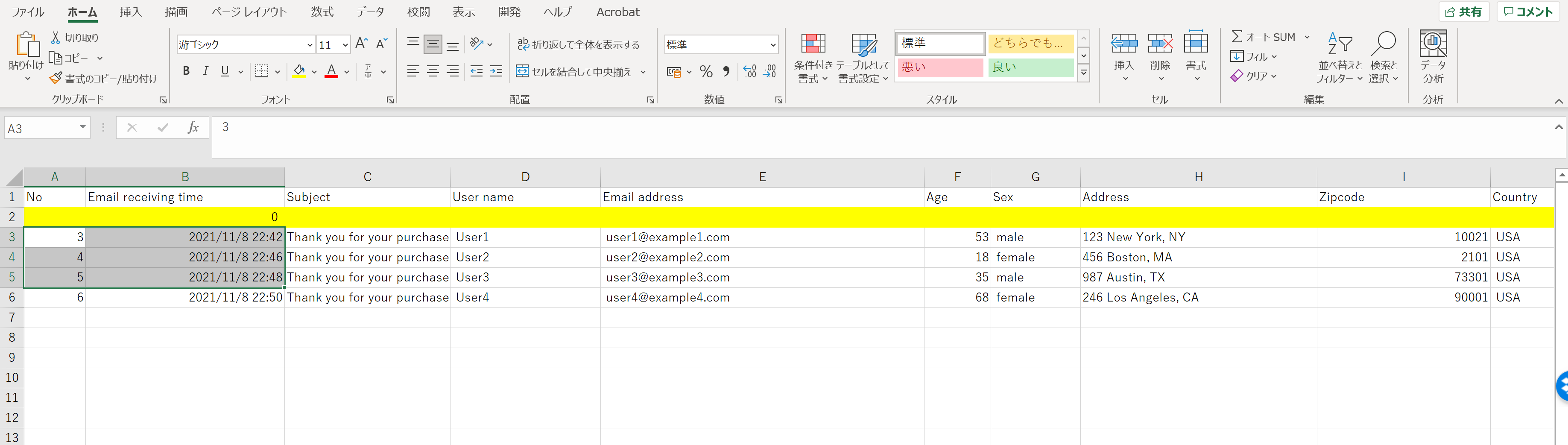}Instructions
##### email2excelVBA

Porocess various emails (purchase or inquiry)
Automatic Excel sheet generation
Update incoming emails from a specific OutLook account
Simple to use

How to use``````
Sub email2excel_en()

Dim ol_obj_df, f, i, j, k, n As Long
Dim ol_obj, Accounts, acc As Object
Dim ol_obj_ns, ol_obj_item As Object

Dim bodywords As String
Dim arr() As String

Dim lastRow As Long
Dim lastTime As Double

lastRow = Cells(Rows.Count, 2).End(xlUp).Row
lastTime = Cells(Rows.Count, 2).End(xlUp).Value
'MsgBox lastTime
k = lastRow

' To create an Outlook object (ol_obj)
Set ol_obj = CreateObject("Outlook.Application")

'To select email account
Set Accounts = ol_obj.Session.Accounts
For Each acc In Accounts
'put an email address of interest below
If acc = "shishid@saaipf.com" Then
Set f = acc.DeliveryStore.GetDefaultFolder(6)
For i = 1 To f.Items.Count
Set ol_obj_item = f.Items(i)
'update incoming emails of interest
'put the "subject" of email to be incorporated
If ol_obj_item.Subject = "Thank you for your purchase of bFaaaP Switch" Then
k = k + 1

Cells(k, 1) = k
Cells(k, 3) = ol_obj_item.Subject

Cells(k, 21) = ol_obj_item.Body

bodywords = Cells(k, 21).Value
'split the body of emial by CRLF
arr = Split(bodywords, vbCrLf)
'To process each line
For j = LBound(arr) To UBound(arr)
If InStr(arr(j), "Name:") <> 0 Then
Cells(k, 4) = GiveContent(arr(j), "Name:")
End If
If InStr(arr(j), "Email:") <> 0 Then
Cells(k, 5) = GiveContent(arr(j), "Email:")
End If
If InStr(arr(j), "Age:") <> 0 Then
Cells(k, 6) = GiveContent(arr(j), "Age:")
End If
If InStr(arr(j), "Sex:") <> 0 Then
Cells(k, 7) = GiveContent(arr(j), "Sex:")
End If
If InStr(arr(j), "Address: ") <> 0 Then
End If
If InStr(arr(j), "Zipcode:") <> 0 Then
Cells(k, 9) = GiveContent(arr(j), "Zipcode:")
End If
If InStr(arr(j), "Country:") <> 0 Then
Cells(k, 10) = GiveContent(arr(j), "Country:")
End If

If InStr(arr(j), "Message:") <> 0 Then
Cells(k, 11) = GiveContent(arr(j), "Message:")
End If
Next j
End If
End If
Next
End If
Next
'To prohibit folding of each cell
Cells.WrapText = False
End Sub

Function GiveContent(stringline As String, markerword As String) As String
Dim processedContent As String
Dim markerwordcount As Long
markerwordcount = Len(markerword)

processedContent = Mid(stringline, InStr(stringline, markerword) + markerwordcount)
'If the stringline ends with ",", remove it
If Right(processedContent, 1) = "," Then
processedContent = Left(processedContent, Len(processedContent) - 1)
End If

GiveContent = processedContent

End Function

``````

## Introduction

Here is the situation. You receive a lot of incomming purchase emails sent from a server on AWS.Now, you want a client information list ready in an Excel worksheet like below.This email2excelVBA can automatically achieve the job by just one click.

## How to change and set

1. Replace the sample email address by your Outlook acount of interest.

``````
'put an email address of interest below
If acc = "shishid@saaipf.com" Then
``````

2. Change the subject of email to your own one.

``````
'put the "subject" of email to be incorporated
If ol_obj_item.Subject = "Thank you for your purchase of bFaaaP Switch" Then
``````

3. Select and replace each marker word of the email body (sepalated by CRLF) (e.g., "Name:").

``````
If InStr(arr(j), "Name:") <> 0 Then
Cells(k, 4) = GiveContent(arr(j), "Name:")
End If
If InStr(arr(j), "Email:") <> 0 Then
Cells(k, 5) = GiveContent(arr(j), "Email:")
End If
If InStr(arr(j), "Age:") <> 0 Then
Cells(k, 6) = GiveContent(arr(j), "Age:")
End If
If InStr(arr(j), "Sex:") <> 0 Then
Cells(k, 7) = GiveContent(arr(j), "Sex:")
End If
If InStr(arr(j), "Address: ") <> 0 Then
End If
If InStr(arr(j), "Zipcode:") <> 0 Then
Cells(k, 9) = GiveContent(arr(j), "Zipcode:")
End If
If InStr(arr(j), "Country:") <> 0 Then
Cells(k, 10) = GiveContent(arr(j), "Country:")
End If

If InStr(arr(j), "Message:") <> 0 Then
Cells(k, 11) = GiveContent(arr(j), "Message:")
End If
``````

4. Run the macro (cell(B,2) should be 0 to incorporate incoming emails in the ascending order).
See the top video for specific instructions.

5. You may contact Tomo at shishid@saaipf.com